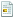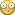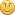January 20, 2022, 06:20:33 AM

News:

Own IWBasic 2.x ? -----> Get your free upgrade to 3.x now.........

Puzzlet #38

Started by GWS, March 28, 2014, 08:57:49 PM

0 Members and 1 Guest are viewing this topic.

GWSMarch 28, 2014, 08:57:49 PM
Here's another maths puzzle by Dave Ellis from 2003 ..

This one is to find a 5-digit integer whose value is equal to the sum of the factorials
of its own digits.declare SumFactorials(s: int)
declare Factorial (f: int)
declare PrintResult()
def flag, n, sumFact: int
openconsole
print "Searching ...": print
n = 10000
flag = 0

do
sumFact = SumFactorials(n)
if sumFact = n
flag = 1
else
n = n + 1
endif
until flag

PrintResult()
print: print "Press any key to exit ..."
do: until inkey\$ <> ""
closeconsole
end

sub SumFactorials(s)
' returns the sum of the factorials of
' the individual digits of s
def i, num, sum: int
num = s
sum = 0
for i = 1 to 5
sum = sum + Factorial(num % 10)
num = int(num/10)
next i
return sum

sub Factorial(f)
' returns the factorial of f
def it, fact: int
fact = 1
for it = 1 to f
fact = fact*it
next it
return fact

sub PrintResult
' pretty printer
def i, digit: int
def n\$: string
n\$ = str\$(n)
for i = 2 to 6
digit = val(mid\$(n\$, i, 1))
print mid\$(n\$,i,1) + "! = ",
print using ("########", Factorial(digit))
next i
print: print "Sum: ",
print using ("########", n)
return

Hope you find these little puzzles interesting ..Graham
Tomorrow may be too late ..

LarryMcMarch 28, 2014, 09:43:37 PM #1
nifty little brain teasers.
LarryMc
Larry McCaughnAuthor of IWB+, Custom Button Designer library, Custom Chart Designer library, Snippet Manager, IWGrid control library, LM_Image control library